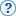freesilentMaskedMerge的问题```AA = taa.TAAmbk(video, aatype = -2, sharp = 0.7, aarepair = 24) AAimg = core.imwri.Read(r'd:\download\test\new\%05d.png', firstnum = 00000) AAtoy = mvf.ToYUV(AAimg, matrix = mat, css = "22", full = 1) AAmask = core.std.AssumeFPS(clip = AAtoy, src = video) AA5 = core.std.MaskedMerge(clipa = raw, clipb = AA, mask = AAmask, first_plane = 1) . . . . combine_video = ......+ AA5.std.trim(first = 144, last = 263) +...... ```

```AAvideo = video.std.Trim(first = 11312, last = 11623) AAsource = taa.TAAmbk(AAvideo, aatype = -2, sharp = 0.7, aarepair = 24) AAimg = core.imwri.Read(r'd:\download\test\new\%05d.png', firstnum = 00000) AAtoy = mvf.ToYUV(AAimg, matrix = mat, css = "22", full = 1) AAmask = core.std.AssumeFPS(clip = AAtoy, src = video) AA5 = core.std.MaskedMerge(clipa = AAvideo, clipb = AAsource, mask = AAmask, first_plane = 1) . . . . combine_video = ......+ AA5 + ...... ```raw是11312~11623帧，南极人第8集。